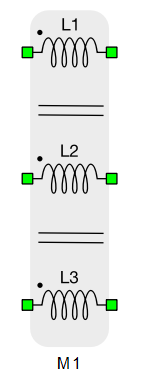# Three Coupled Inductors

This section describes three coupled inductors.

Three coupled inductors are implemented as inductors and voltage controlled voltage sources.

Table 1. Three coupled inductors component in HIL Toolbox
component component dialog window component parametersThree coupled inductors• General
• ${L}_{1}$ - inductance
• ${I}_{\mathrm{1_0}}$ - initial current
• ${R}_{1}$ - resistance
• ${L}_{2}$ - inductance
• ${I}_{\mathrm{2_0}}$ - initial current
• ${R}_{2}$ - resistance
• ${L}_{3}$ - inductance
• ${I}_{\mathrm{3_0}}$ - initial current
• ${R}_{3}$ - resistance
• ${k}_{\mathrm{12}}$ - ${L}_{1}$ and ${L}_{2}$ coupling coefficients
• ${k}_{\mathrm{13}}$ - ${L}_{1}$ and ${L}_{3}$ coupling coefficients
• ${k}_{\mathrm{23}}$ - ${L}_{2}$ and ${L}_{3}$ coupling coefficients

## Description

Three coupled inductors are represented by matrix $\left[\begin{array}{ccc}L& M& M\\ M& L& M\\ M& M& L\end{array}\right]$ where off diagonal elements are calculated as ${M}_{ij}={M}_{ji}={k}_{ij}*\sqrt{{L}_{i}*{L}_{j}}$